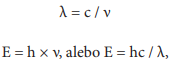# INTRODUCTION

The electromagnetic spectrum (EM) is the whole range of electromagnetic radiation. The EM radiation can be described as a flow of photons transporting energy, each photon moving with the speed of light as a separate wave. The only difference between radio waves, visible light, X-rays, and other kinds of EM radiation is the photons’ energy. Radio waves are transported by photons with very low energies, the microwaves have slightly higher energies, infrared radiation – higher than microwaves, then come optical waves, UV, X-rays, and gamma rays have the highest energies.

In this section we describe the electromagnetic spectrum (EM), its different ranges, waves and photons. We discuss the atmospheric windows, i.e. wavelengths at which electromagnetic radiation penetrates the Earth's atmosphere. We include some practical exercises for students from different age groups, aiming to illustrate and help better understand the EM spectrum and its parts, the scientific methods and experiments and to teach the students how to prepare, conduct and present the results from an experiment.

# An introduction to the electromagnetic spectrum

Depending on the energy a photon carries, it behaves either as a wave (at low energies), or as a particle (at high energies). This is a manifestation of the “wave-particle duality” of light (the term comes from quantum mechanics) – the photons are both waves and particles simultaneously. The photons act either as one or the other depending on the physical conditions. The wave-particle duality is most pronounced among the particles. The principle can be true for bigger objects, but the bigger the object, the fainter its wave properties show. WE can not speak about physical differences between the different types of EM radiation. - there are none, just different behaviour, entirely due to the photons’ energy.

The electromagnetic spectrum can be expressed through either of the following three physical parameters: the frequency (denoted by ν), the wavelength (λ), or the photon energy (E).

These three are interconnected:where c is the speed of light in vacuum c = 299 792,458 m/s or about 300 000 km/s, h is the Planck's constant, h = 6,626 × 10−34 J · s = 4.136 × 10−15 eV · s.

Both Plank’s constant and the speed of light are constants, which means that their value does not change in any circumstances.

Figure 1 shows the connection between wavelength and frequency for different spectral ranges, and the conversion between frequency (denoted by ν), wavelength (λ), and photon energy (E) is shown in Figure 2.

In astronomy, the observed frequencies range from about 2.4 × 1023 Hz (gamma-rays with energy of 1 GeV from supernova explosions) to about 1 kHz (radio waves with energy 4×10-12 eV from the ionised interstellar medium). Gamma-rays have very short wavelengths – of the order of or smaller than the size of a single atom. At the other end, the longest wavelengths can be comparable to the sizes of the stars (in theory the limit is the size of the Universe!).

Whenever electromagnetic waves exist in a medium with matter, their wavelength is decreased. Wavelengths of electromagnetic radiation, no matter what medium they are traveling through, are usually quoted in terms of the vacuum wavelength, although this is not always explicitly stated.Fig. 1 Connection between wavelength and frequency for different spectral rangesFig. 2 Conversion between frequency (denoted by ν), wavelength (λ), and photon energy (E)

filter_none